# Which of the following would increase the capacitance of a parallel-plate capacitor? I. Insert a dielectric...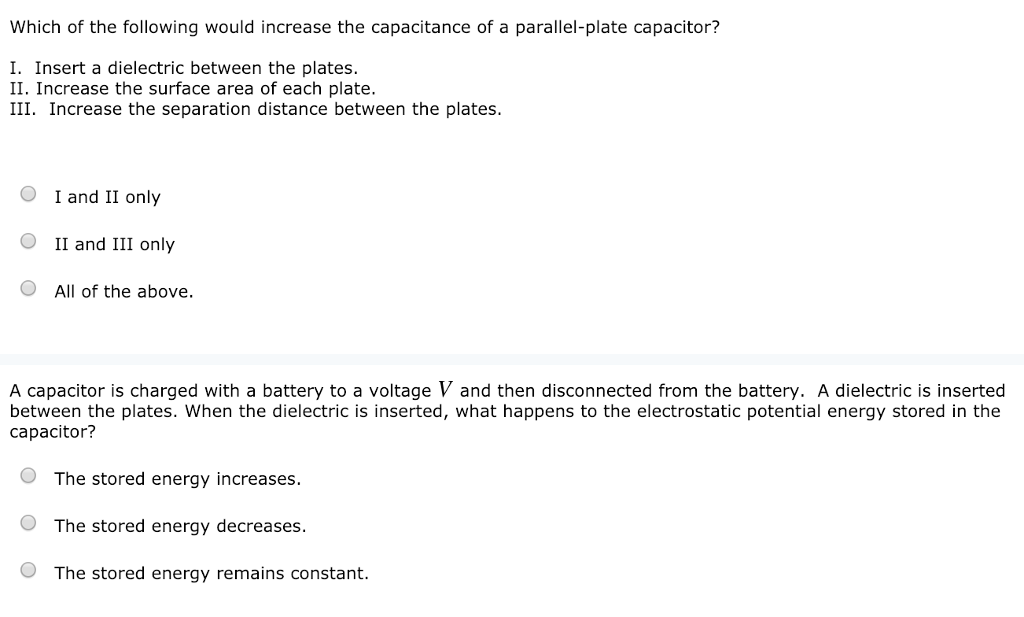Which of the following would increase the capacitance of a parallel-plate capacitor? I. Insert a dielectric between the plates. II. Increase the surface area of each plate. III. Increase the separation distance between the plates. O I and II only OII and III only All of the above. A capacitor is charged with a battery to a voltage V and then disconnected from the battery. A dielectric is inserted between the plates. When the dielectric is inserted, what happens to the electrostatic potential energy stored in the capacitor? O The stored energy increases. O The stored energy decreases. The stored energy remains constant.

1) correct option is I and II only

we know the relation

C=koA/d

so if we increase the surface area it increases the capacitance.

when we introduce a dielectric between the plates of the capacitor the charge in the capacitor increases because the dielectric creates its own electric field due to polarization

and since C=q/v

capacitance increases.

2) the correct option is -the stored energy decreases.

the energy in the capacitor is given U=Q2/2C

as we have discussed in previous question, when dielectric is inserted then the capacitance increases which decreses the potential energy as capacitance is inversely proportional to potential energy.

#### Earn Coin

Coins can be redeemed for fabulous gifts.

Similar Homework Help Questions
• ### (1 point) A parallel plate capacitor is filled with a dielectric that has a dielectric constant...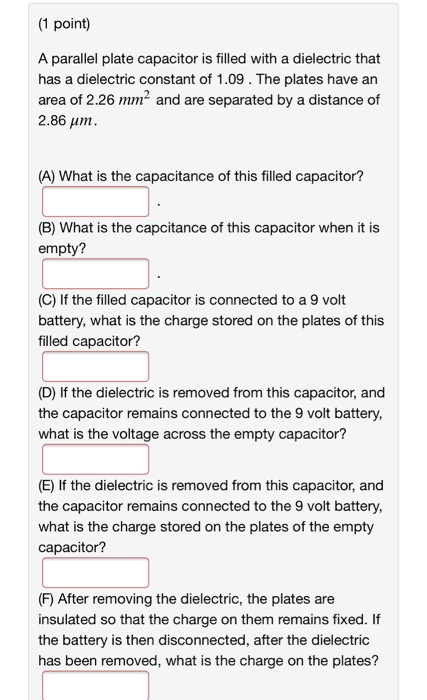(1 point) A parallel plate capacitor is filled with a dielectric that has a dielectric constant of 1.09. The plates have an area of 2.26 mm² and are separated by a distance of 2.86 um. (A) What is the capacitance of this filled capacitor? (B) What is the capcitance of this capacitor when it is empty? (C) If the filled capacitor is connected to a 9 volt battery, what is the charge stored on the plates of this filled capacitor?...

• ### A parallel-plate capacitor has capacitance 7.50 ?F. (a) How much energy is stored in the capacitor...

A parallel-plate capacitor has capacitance 7.50 ?F. (a) How much energy is stored in the capacitor if it is connected to a 15.00-V battery? ?J (b) If the battery is disconnected and the distance between the charged plates doubled, what is the energy stored? ?J (c) The battery is subsequently reattached to the capacitor, but the plate separation remains as in part (b). How much energy is stored? ?J

• ### A parallel-plate capacitor has capacitance 7.50 uF. (a) How much energy is stored in the capacitor...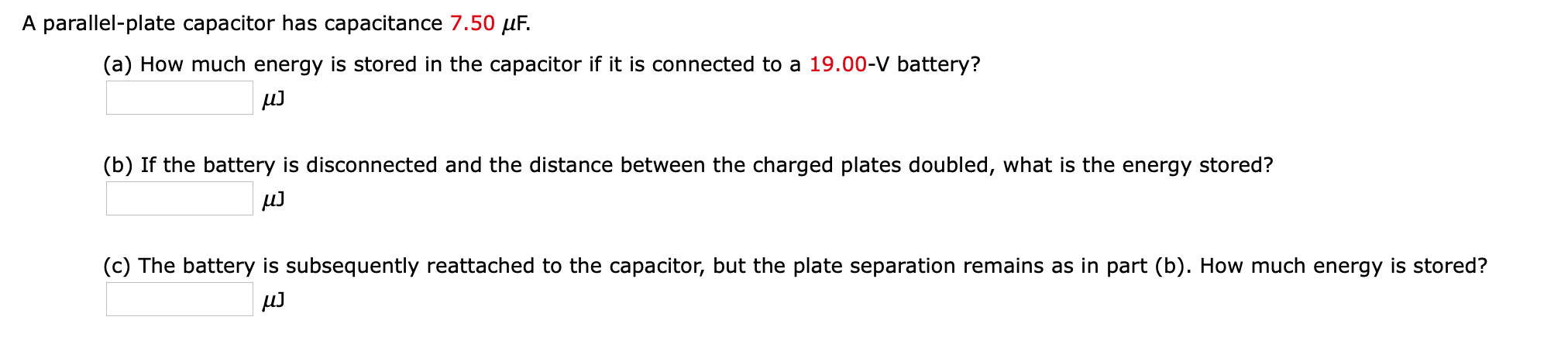A parallel-plate capacitor has capacitance 7.50 uF. (a) How much energy is stored in the capacitor if it is connected to a 19.00-V battery? ա․ (b) If the battery is disconnected and the distance between the charged plates doubled, what is the energy stored? uJ (c) The battery is subsequently reattached to the capacitor, but the plate separation remains as in part (b). How much energy is stored? u]

• ### A parallel-plate capacitor has capacitance 5.50 μF. (a) How much energy is stored in the capacitor...

A parallel-plate capacitor has capacitance 5.50 μF. (a) How much energy is stored in the capacitor if it is connected to a 18.00-V battery? ______μJ (b) If the battery is disconnected and the distance between the charged plates doubled, what is the energy stored? _______μJ (c) The battery is subsequently reattached to the capacitor, but the plate separation remains as in part (b). How much energy is stored? _______μJ

• ### A parallel plate capacitor with plate separation d is connected to a battery. The capacitor is...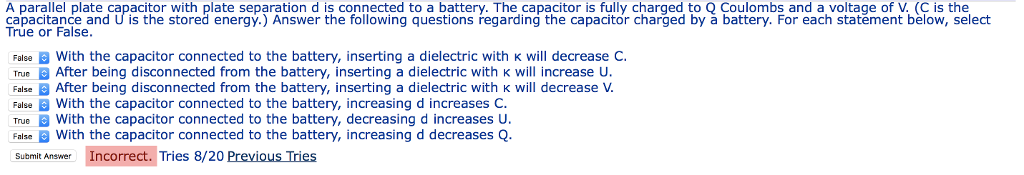A parallel plate capacitor with plate separation d is connected to a battery. The capacitor is fully charged to Q Coulombs and a voltage of V. (C is the capacitance and U is the stored energy.) Answer the following questions regarding the capacitor charged by a battery. For each statement below, select True or False. With the capacitor connected to the battery, inserting a dielectric with κ will decrease C. After being disconnected from the battery, inserting a dielectric with...

• ### A parallel plate capacitor with plate separation d is connected to a battery. The capacitor is...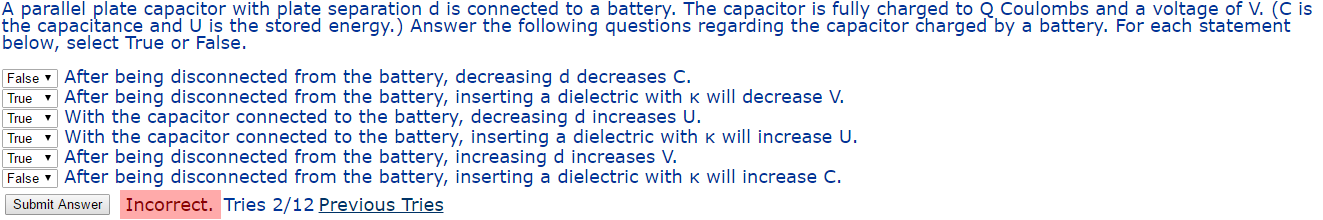A parallel plate capacitor with plate separation d is connected to a battery. The capacitor is fully charged to Q Coulombs and a voltage of V. (C is the capacitance and U is the stored energy.) Answer the following questions regarding the capacitor charged by a battery. For each statement below, select True or False. After being disconnected from the battery, decreasing d decreases C. After being disconnected from the battery, inserting a dielectric with k will decrease V. With...

• ### A parallel-plate capacitor has capacitance 9.50 uF. (a) How much energy is stored in the capacitor...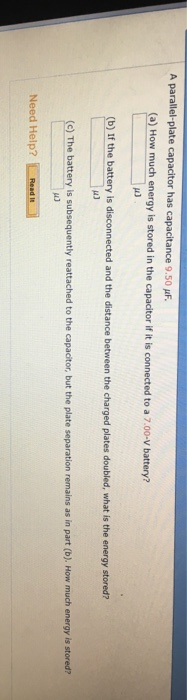A parallel-plate capacitor has capacitance 9.50 uF. (a) How much energy is stored in the capacitor if it is connected to a 7.00-V battery? (b) If the battery is disconnected and the distance between the charged plates doubled, what is the energy stored? (c) The battery is subsequently reattached to the capacitor, but the plate separation remains as in part (b). How much energy is stored? Need Help? Read It 13. -120 points OSColPhys1 19.5.064 XP A large capacitance of...

• ### A parallel plate capacitor with plate separation d is connected to a battery. The capacitor is...

A parallel plate capacitor with plate separation d is connected to a battery. The capacitor is fully charged to Q Coulombs and a voltage of V. (C is the capacitance and U is the stored energy.) Answer the following questions regarding the capacitor charged by a battery. For each statement below, select True or False. With the capacitor connected to the battery, inserting a dielectric with κ will increase C. After being disconnected from the battery, inserting a dielectric with...

• ### A parallel plate capacitor with plate separation d is connected to a battery. The capacitor is...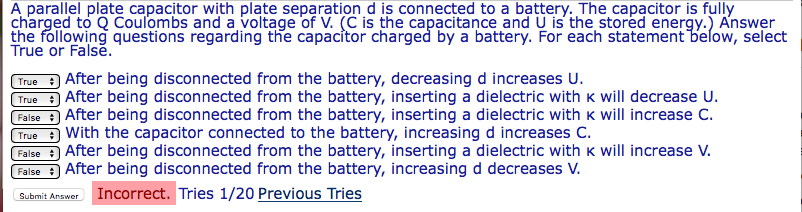A parallel plate capacitor with plate separation d is connected to a battery. The capacitor is fully charged to O Coulombs and a voltage of V. (C is the capacitance and U is the stored energy.) Answer the following questions regarding the capacitor charged by a battery. For each statement below, select True or Falsě. True After being disconnected from the battery, decreasing d increases U. True After being disconnected from the battery, inserting a dielectric with k will decrease...

• ### A parallel plate capacitor with plate separation d is connected to a battery. The capacitor is...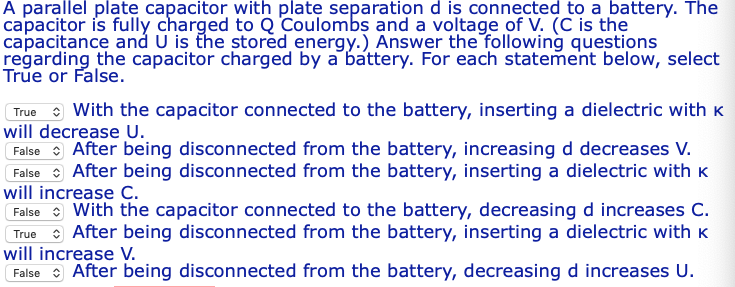A parallel plate capacitor with plate separation d is connected to a battery. The capacitor is fully charged to Q Coulombs and a voltage of V. (C is the capacitance and U is the stored energy.) Answer the following questions regarding the capacitor charged by a battery. For each statement below, select True or False TrueWith the capacitor connected to the battery, inserting a dielectric withK will decrease U. False After being disconnected from the battery, increasing d decreases V...